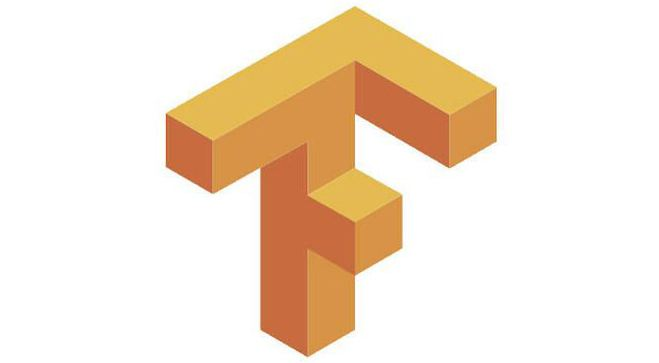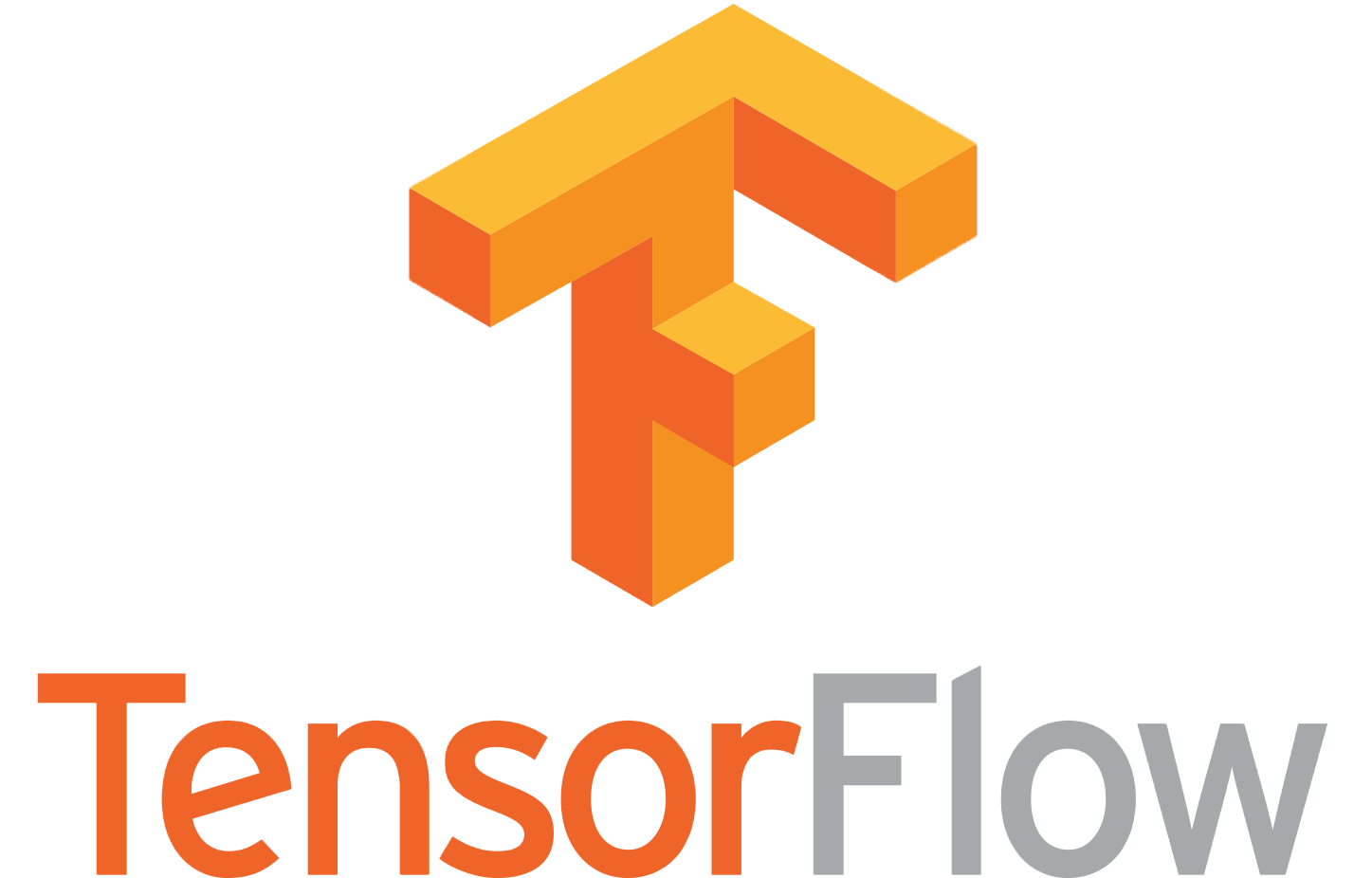# 在TensorFlow中实现矩阵维度扩展_pythonfoo = tf.constant([[1,2,3], [4,5,6], [7,8,9]])print(foo[tf.newaxis, :, :].eval()) # => [[[1,2,3], [4,5,6], [7,8,9]]]print(foo[:, tf.newaxis, :].eval()) # => [[[1,2,3]], [[4,5,6]], [[7,8,9]]]print(foo[:, :, tf.newaxis].eval()) # => [[,,], [,,],[,,]]tf.expand_dims()

tf.squeeze()

tf.expand_dims()

tf.expand_dims(input, axis=None, name=None, dim=None)

# 't' is a tensor of shape shape(expand_dims(t, 0)) ==> [1, 2]shape(expand_dims(t, 1)) ==> [2, 1]shape(expand_dims(t, -1)) ==> [2, 1]# 't2' is a tensor of shape [2, 3, 5]shape(expand_dims(t2, 0)) ==> [1, 2, 3, 5]shape(expand_dims(t2, 2)) ==> [2, 3, 1, 5]shape(expand_dims(t2, 3)) ==> [2, 3, 5, 1]

tf.squeeze()

tf.squeeze(input, axis=None, name=None, squeeze_dims=None)

# 't' is a tensor of shape [1, 2, 1, 3, 1, 1] shape(squeeze(t)) ==> [2, 3]# 't' is a tensor of shape [1, 2, 1, 3, 1, 1] shape(squeeze(t, [2, 4])) ==> [1, 2, 3, 1]

Ubuntu安装tensorflow先安装python-dev，再安装tensorflow就好了sudo apt-get install python-devpip install内容来自www.zgxue.com请勿采集。

• 本文相关：
• tensorflow tf.tile()的用法实例分析
• tensorflow实现将标签变为one-hot形式
• tensorflow卷积实现原理+手写python代码实现卷积教程
• 基于tensorflow一维卷积用法详解
• 多版本python的pip 升级后, pip2 pip3 与python版本失配解决方法
• python实现windows和linux之间互相传输文件(文件夹)的方法
• python实现监控linux性能及进程消耗性能的方法
• python随机生成彩票号码的方法
• 提升python处理速度原理及方法实例
• python django框架实现应用添加logging日志操作示例
• python实现网站文件的全备份和差异备份
• 在python 中实现运行多条shell命令
• python while循环使用else语句代码实例
• python 正则表达式爬虫使用案例解析
• tensor flow 用什么方式安装好
• 安装tensor flow后怎么配置环境
• 液力变矩器是如何变矩的
• 工程力学中二矩式与三矩式的区别
• 什么是静矩
• pythonista3能安装tensor flow吗？
• 什么是一阶矩和二阶矩？
• 变矩器的作用
• 如何利用机器学习最火的tensor flow架构预测房价
• 中规中矩 近义词
• 免责声明 - 关于我们 - 联系我们 - 广告联系 - 友情链接 - 帮助中心 - 频道导航